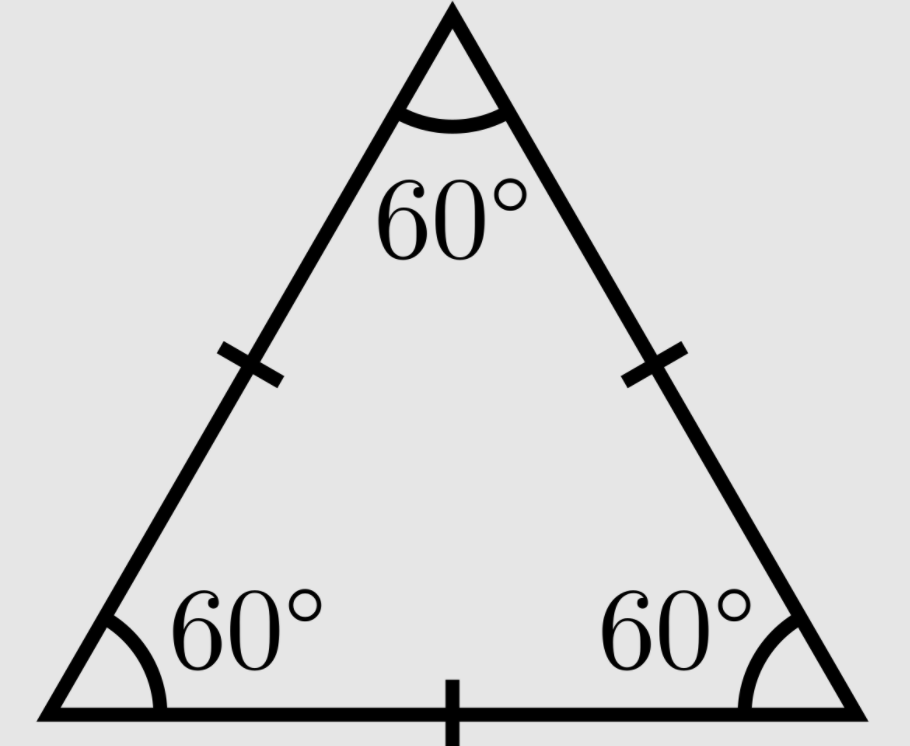100

36x9=

324

100

How many sides does a quadrilateral have?

4

100

3/6 + 2/6=

5/6

100

What part of a division problem is the answer?

quotient

100

What type of angle is this angle?Obtuse

100

How many minutes are in 1 hour and 25 minutes?

85 minutes

200

324 x 6=

1,944

200

What is this triangle called?Equilateral Triangle

200

2/3 = ?/12

8

200

96 divide 8

12
200

Colin had 118 basketball cards.  Dylan had 124 basketball cards. Then he lost 7 of them.  How many total cards do the boys have now?

200

4 feet= ___ inches

48 inches

300

8,251 x 9=

74,259

300

What type of angle is 90 degrees?

right angle

300

Which of the following fractions is EQUIVALENT to 2/3?

3/4

6/9

5/6

6/9

6/9

300

34 divide 6 =

5 R 4

300

What do you call an answer to a multiplication equation.

The Product.

300

10 yards ______  30 feet

> greater than

< less than

= equal

= Equal

400

13 X 85=

1,105

400

A square has one side that is 12 cm long.  What is the PERIMETER of the square?

48 cm

400

3 5/7 + 6 2/7=

7

400

124 divide 4 =

31

400

4,027-2,738=?

1,289

400

Which is more 3 feet or 42 inches?

42 inches

600

8653 x 9 =

77,877

600

A square has one side that is 12 cm long.  What is the AREA of the square?

144 square cm

600

Mrs. Ruche drank 1 2/4 liters of water in the morning. Then after lunch she drank 1 3/4 more liters of water. how much water did Mrs. Ruche drink?

3 1/4

600

528 divide 5 =

105 R 3

600

ORDER OF OPERATION: PEMDAS

12x5-(6x8) =

12

600

Jessenia's backpack weighs 5 lbs. Karla's backpack weighs 9 lbs.  How many more OUNCES is Karla's backpack than Jessenia's?

1 lb = 16 oz

Karla's backpack weighs 64 oz more.

Click to zoom